# SL-2.pptx

Oct. 30, 2022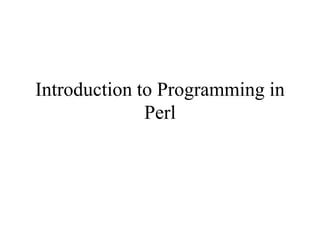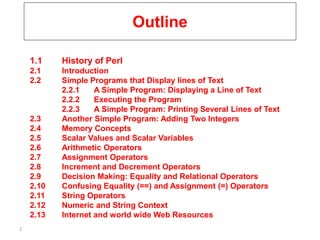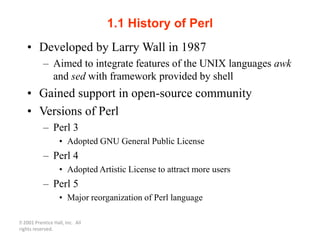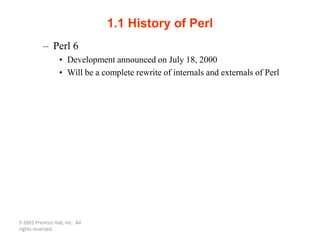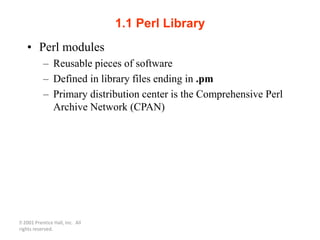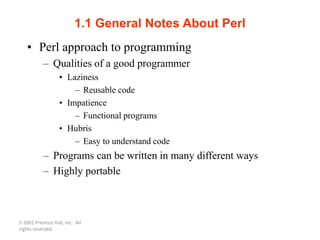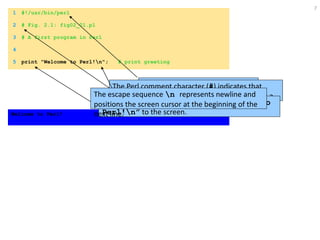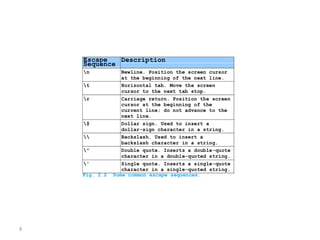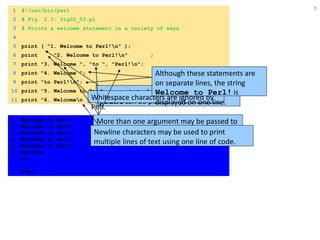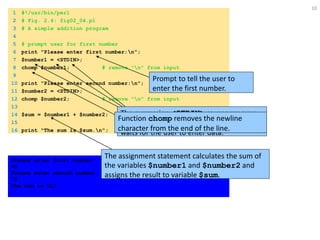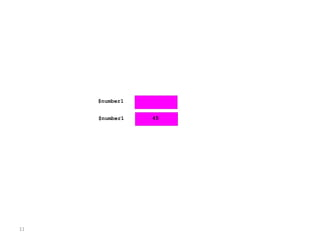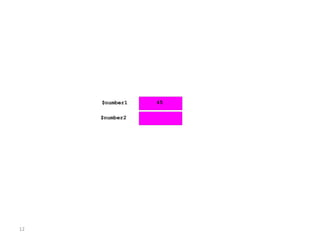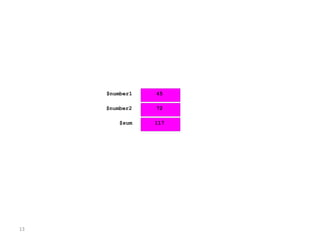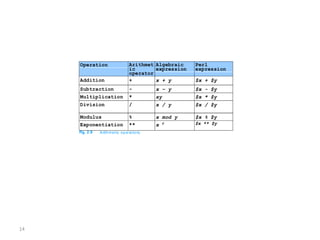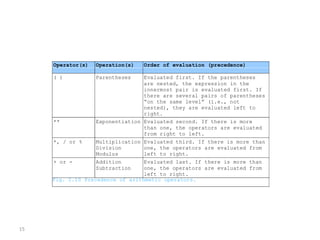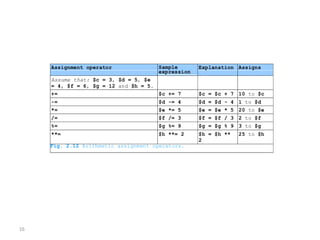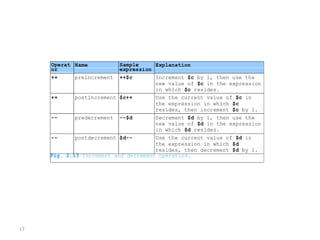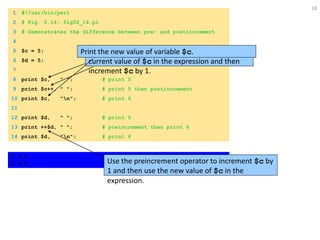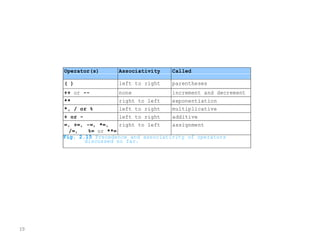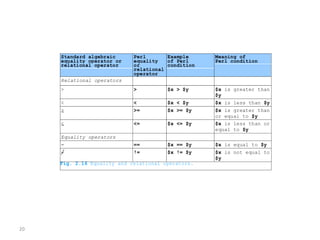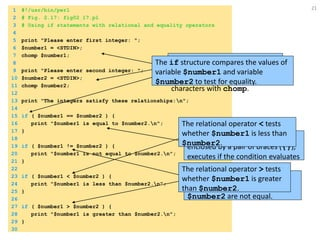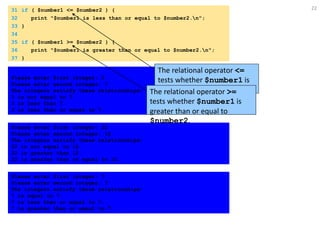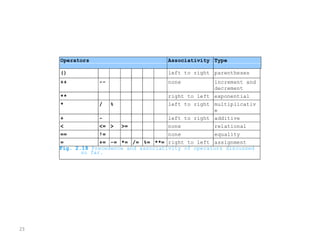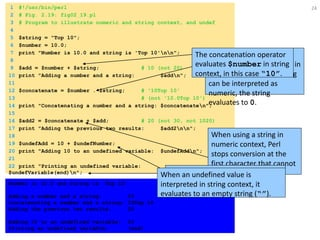1 of 24

### SL-2.pptx

• 2. 2 Outline 1.1 History of Perl 2.1 Introduction 2.2 Simple Programs that Display lines of Text 2.2.1 A Simple Program: Displaying a Line of Text 2.2.2 Executing the Program 2.2.3 A Simple Program: Printing Several Lines of Text 2.3 Another Simple Program: Adding Two Integers 2.4 Memory Concepts 2.5 Scalar Values and Scalar Variables 2.6 Arithmetic Operators 2.7 Assignment Operators 2.8 Increment and Decrement Operators 2.9 Decision Making: Equality and Relational Operators 2.10 Confusing Equality (==) and Assignment (=) Operators 2.11 String Operators 2.12 Numeric and String Context 2.13 Internet and world wide Web Resources
• 3. 2001 Prentice Hall, Inc. All rights reserved. 1.1 History of Perl • Developed by Larry Wall in 1987 – Aimed to integrate features of the UNIX languages awk and sed with framework provided by shell • Gained support in open-source community • Versions of Perl – Perl 3 • Adopted GNU General Public License – Perl 4 • Adopted Artistic License to attract more users – Perl 5 • Major reorganization of Perl language
• 4. 2001 Prentice Hall, Inc. All rights reserved. 1.1 History of Perl – Perl 6 • Development announced on July 18, 2000 • Will be a complete rewrite of internals and externals of Perl
• 5. 2001 Prentice Hall, Inc. All rights reserved. 1.1 Perl Library • Perl modules – Reusable pieces of software – Defined in library files ending in .pm – Primary distribution center is the Comprehensive Perl Archive Network (CPAN)
• 6. 2001 Prentice Hall, Inc. All rights reserved. 1.1 General Notes About Perl • Perl approach to programming – Qualities of a good programmer • Laziness – Reusable code • Impatience – Functional programs • Hubris – Easy to understand code – Programs can be written in many different ways – Highly portable
• 7. 7 1 #!/usr/bin/perl 2 # Fig. 2.1: fig02_01.pl 3 # A first program in Perl 4 5 print "Welcome to Perl!n"; # print greeting Welcome to Perl! The “shebang” construct (#!) indicates the path to the Perl interpreter on Unix and Linux systems. The Perl comment character (#) indicates that everything on the current line following the # is a comment. Function print outputs the string “Welcome to Perl!n” to the screen. The escape sequence n represents newline and positions the screen cursor at the beginning of the next line.
• 8. 8 Escape Sequence Description n Newline. Position the screen cursor at the beginning of the next line. t Horizontal tab. Move the screen cursor to the next tab stop. r Carriage return. Position the screen cursor at the beginning of the current line; do not advance to the next line. \$ Dollar sign. Used to insert a dollar-sign character in a string. Backslash. Used to insert a backslash character in a string. " Double quote. Inserts a double-quote character in a double-quoted string. ' Single quote. Inserts a single-quote character in a single-quoted string. Fig. 2.2 Some common escape sequences.
• 9. 9 1 #!/usr/bin/perl 2 # Fig. 2.3: fig02_03.pl 3 # Prints a welcome statement in a variety of ways 4 5 print ( "1. Welcome to Perl!n" ); 6 print "2. Welcome to Perl!n" ; 7 print "3. Welcome ", "to ", "Perl!n"; 8 print "4. Welcome "; 9 print "to Perl!n"; 10 print "5. Welcome to Perl!n"; 11 print "6. Welcomen tonn Perl!n"; 1. Welcome to Perl! 2. Welcome to Perl! 3. Welcome to Perl! 4. Welcome to Perl! 5. Welcome to Perl! 6. Welcome to Perl! Arguments to built-in functions like print can be placed in parentheses. Whitespace characters are ignored by Perl. More than one argument may be passed to function print. Although these statements are on separate lines, the string Welcome to Perl! is displayed on one line. Newline characters may be used to print multiple lines of text using one line of code.
• 10. 10 1 #!/usr/bin/perl 2 # Fig. 2.4: fig02_04.pl 3 # A simple addition program 4 5 # prompt user for first number 6 print "Please enter first number:n"; 7 \$number1 = <STDIN>; 8 chomp \$number1; # remove "n" from input 9 10 print "Please enter second number:n"; 11 \$number2 = <STDIN>; 12 chomp \$number2; # remove "n" from input 13 14 \$sum = \$number1 + \$number2; 15 16 print "The sum is \$sum.n"; Please enter first number: 45 Please enter second number: 72 The sum is 117. Prompt to tell the user to enter the first number. Declare scalar variable \$number1. Assignment operator =. The expression <STDIN> causes program execution to pause while the computer waits for the user to enter data. Function chomp removes the newline character from the end of the line. The assignment statement calculates the sum of the variables \$number1 and \$number2 and assigns the result to variable \$sum.
• 14. 14 Operation Arithmet ic operator Algebraic expression Perl expression Addition + x + y \$x + \$y Subtraction - x – y \$x - \$y Multiplication * xy \$x * \$y Division / x / y \$x / \$y Modulus % x mod y \$x % \$y Exponentiation ** x y \$x ** \$y Fig. 2.9 Arithmetic op era tors.
• 15. 15 Operator(s) Operation(s) Order of evaluation (precedence) ( ) Parentheses Evaluated first. If the parentheses are nested, the expression in the innermost pair is evaluated first. If there are several pairs of parentheses “on the same level” (i.e., not nested), they are evaluated left to right. ** Exponentiation Evaluated second. If there is more than one, the operators are evaluated from right to left. *, / or % Multiplication Division Modulus Evaluated third. If there is more than one, the operators are evaluated from left to right. + or - Addition Subtraction Evaluated last. If there is more than one, the operators are evaluated from left to right. Fig. 2.10 Precedence of arithmetic operators.
• 16. 16 Assignment operator Sample expression Explanation Assigns Assume that: \$c = 3, \$d = 5, \$e = 4, \$f = 6, \$g = 12 and \$h = 5. += \$c += 7 \$c = \$c + 7 10 to \$c -= \$d -= 4 \$d = \$d - 4 1 to \$d *= \$e *= 5 \$e = \$e * 5 20 to \$e /= \$f /= 3 \$f = \$f / 3 2 to \$f %= \$g %= 9 \$g = \$g % 9 3 to \$g **= \$h **= 2 \$h = \$h ** 2 25 to \$h Fig. 2.12 Arithmetic assignment operators.
• 17. 17 Operat or Name Sample expression Explanation ++ preincrement ++\$c Increment \$c by 1, then use the new value of \$c in the expression in which \$c resides. ++ postincrement \$c++ Use the current value of \$c in the expression in which \$c resides, then increment \$c by 1. -- predecrement --\$d Decrement \$d by 1, then use the new value of \$d in the expression in which \$d resides. -- postdecrement \$d-- Use the current value of \$d in the expression in which \$d resides, then decrement \$d by 1. Fig. 2.13 Increment and decrement operators.
• 18. 18 1 #!/usr/bin/perl 2 # Fig. 2.14: fig02_14.pl 3 # Demonstrates the difference between pre- and postincrement 4 5 \$c = 5; 6 \$d = 5; 7 8 print \$c, " "; # print 5 9 print \$c++, " "; # print 5 then postincrement 10 print \$c, "n"; # print 6 11 12 print \$d, " "; # print 5 13 print ++\$d, " "; # preincrement then print 6 14 print \$d, "n"; # print 6 5 5 6 5 6 6 Print variable \$c. Use the postincrement operator to use the current value of \$c in the expression and then increment \$c by 1. Print the new value of variable \$c. Use the preincrement operator to increment \$c by 1 and then use the new value of \$c in the expression.
• 19. 19 Operator(s) Associativity Called ( ) left to right parentheses ++ or -- none increment and decrement ** right to left exponentiation *, / or % left to right multiplicative + or - left to right additive =, +=, -=, *=, /=, %= or **= right to left assignment Fig. 2.15 Precedence and associativity of operators discussed so far.
• 20. 20 Standard algebraic equality operator or relational operator Perl equality or relational operator Example of Perl condition Meaning of Perl condition Relational operators > > \$x > \$y \$x is greater than \$y < < \$x < \$y \$x is less than \$y > >= \$x >= \$y \$x is greater than or equal to \$y < <= \$x <= \$y \$x is less than or equal to \$y Equality operators = == \$x == \$y \$x is equal to \$y = != \$x != \$y \$x is not equal to \$y Fig. 2.16 Equality and relational operators.
• 21. 21 1 #!/usr/bin/perl 2 # Fig. 2.17: fig02_17.pl 3 # Using if statements with relational and equality operators 4 5 print "Please enter first integer: "; 6 \$number1 = <STDIN>; 7 chomp \$number1; 8 9 print "Please enter second integer: "; 10 \$number2 = <STDIN>; 11 chomp \$number2; 12 13 print "The integers satisfy these relationships:n"; 14 15 if ( \$number1 == \$number2 ) { 16 print "\$number1 is equal to \$number2.n"; 17 } 18 19 if ( \$number1 != \$number2 ) { 20 print "\$number1 is not equal to \$number2.n"; 21 } 22 23 if ( \$number1 < \$number2 ) { 24 print "\$number1 is less than \$number2.n"; 25 } 26 27 if ( \$number1 > \$number2 ) { 28 print "\$number1 is greater than \$number2.n"; 29 } 30 Prompt the user for two numbers, read in the numbers, and remove the newline characters with chomp. The if structure compares the values of variable \$number1 and variable \$number2 to test for equality. The body of the if structure, enclosed by a pair of braces ({}), executes if the condition evaluates to true. The equality operator != tests whether \$number1 and \$number2 are not equal. The relational operator < tests whether \$number1 is less than \$number2. The relational operator > tests whether \$number1 is greater than \$number2.
• 22. 22 31 if ( \$number1 <= \$number2 ) { 32 print "\$number1 is less than or equal to \$number2.n"; 33 } 34 35 if ( \$number1 >= \$number2 ) { 36 print "\$number1 is greater than or equal to \$number2.n"; 37 } Please enter first integer: 3 Please enter second integer: 7 The integers satisfy these relationships: 3 is not equal to 7. 3 is less than 7. 3 is less than or equal to 7. Please enter first integer: 22 Please enter second integer: 12 The integers satisfy these relationships: 22 is not equal to 12. 22 is greater than 12. 22 is greater than or equal to 12. Please enter first integer: 7 Please enter second integer: 7 The integers satisfy these relationships: 7 is equal to 7. 7 is less than or equal to 7. 7 is greater than or equal to 7. The relational operator <= tests whether \$number1 is less than or equal to \$number2. The relational operator >= tests whether \$number1 is greater than or equal to \$number2.
• 23. 23 Operators Associativity Type () left to right parentheses ++ -- none increment and decrement ** right to left exponential * / % left to right multiplicativ e + - left to right additive < <= > >= none relational == != none equality = += -= *= /= %= **= right to left assignment Fig. 2.18 Precedence and associativity of operators discussed so far.